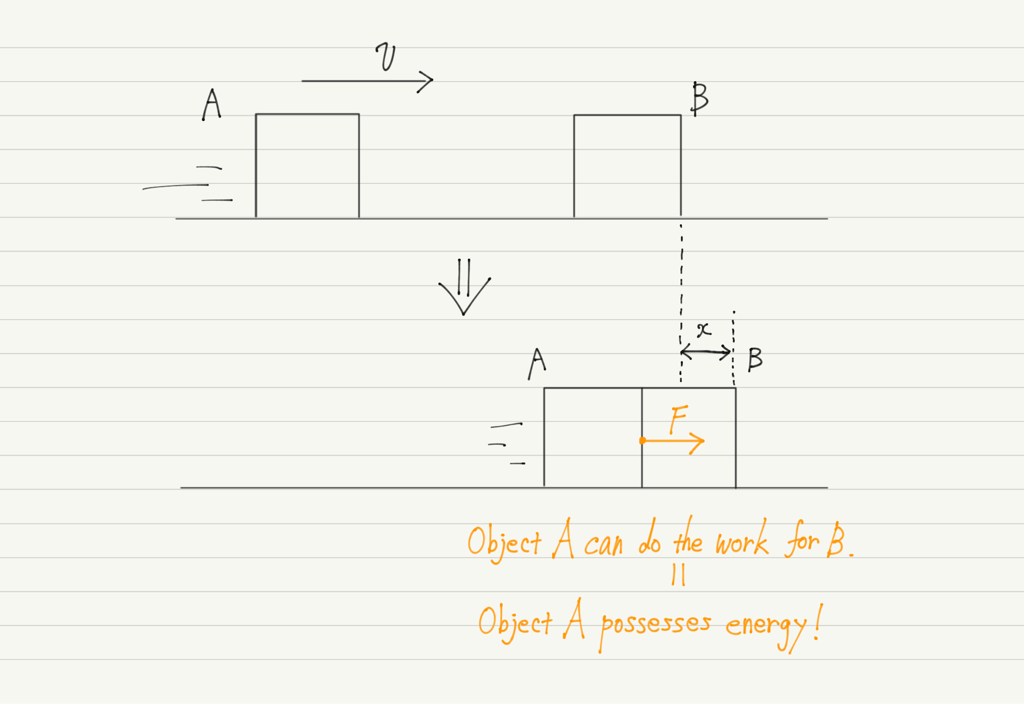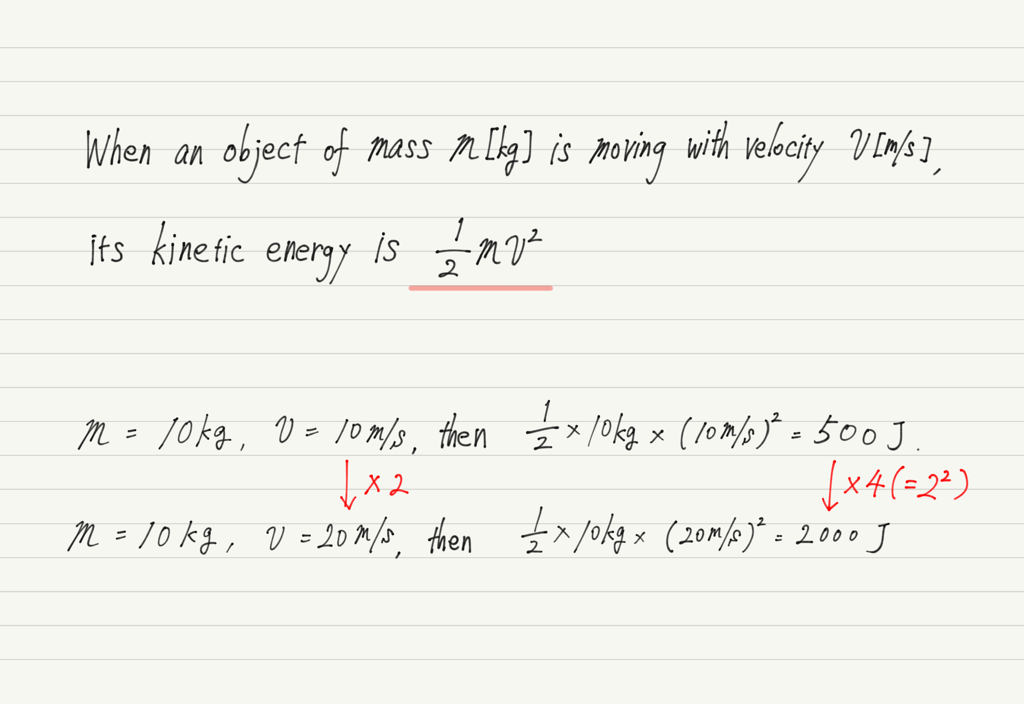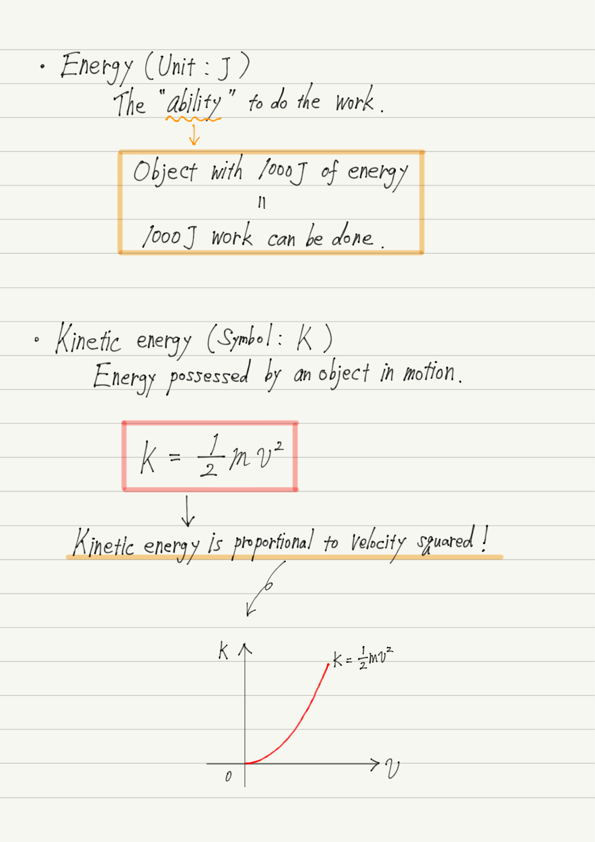# Kinetic Energy

Starting from this lecture, we will learn the calculation of energy.

In mechanics, we study two types of energy, kinetic energy and potential energy, but there are many other types of energy, such as electrical energy, thermal energy, and chemical energy.

By the way, can you explain what “Energy” is?

## Meaning of “Energy”

Most of us have never heard the word “energy,” yet most of us can’t explain what energy is.
Take this opportunity to study up!

Energy, in other words, is “the ability to do work” (of course, “work” in the sense of acting force to move an object).

Because of this close relationship between work and energy, the unit of energy is J (joule), the same as work.

Having 1000J of energy means being able to do 1000J of work. Very simple relationship, isn’t it!

## Energy possessed by an object in motion

When object A in motion hits object B at rest, it pushes B to move (i.e., do work).The energy possessed by an object in motion, such as object A, is called “Kinetic energy”.
The greater the velocity or mass, the greater the kinetic energy.

## How to calculate kinetic energy

With the above explanation alone, we can quickly answer the question,
“If there are two objects with masses of 10 kg and one is moving at 10 m/s and the other at 20 m/s, which has the greater kinetic energy?”

Of course, the 20 m/s object is larger, but we do not know how much larger than the 10 m/s object.
To find out, we need to be able to calculate the kinetic energy!
This will enable us to make numerical comparisons.

Now let’s actually find it by calculation!Turns out that even though the velocity doubled, the kinetic energy did not double!
It is a whopping 4 times as much.

In the past, mechanics often dealt with proportional relationships, but kinetic energy and velocity are not proportional as they are.
Kinetic energy is proportional to the square of velocity!

This is often used in traffic safety lectures to teach the dangers of excessive speed.
Have you ever heard that if you cause an accident at 80 km/h, the damage is four times greater than at 40 km/h, and if you cause an accident at 120 km/h, the damage is nine times greater than at 40 km/h?

The amount of damage is determined by the kinetic energy. Therefore, if an accident occurs at twice the speed, the kinetic energy will be 2 squared (= 4 times), and if an accident occurs at three times the speed, the kinetic energy will be 3 squared (= 9 times).

## Summary of this lecture## Next Time

Next, let’s look at potential energy.

https://www.yukimura-physics.com/entry/dyn-f27

error: Content is protected !!